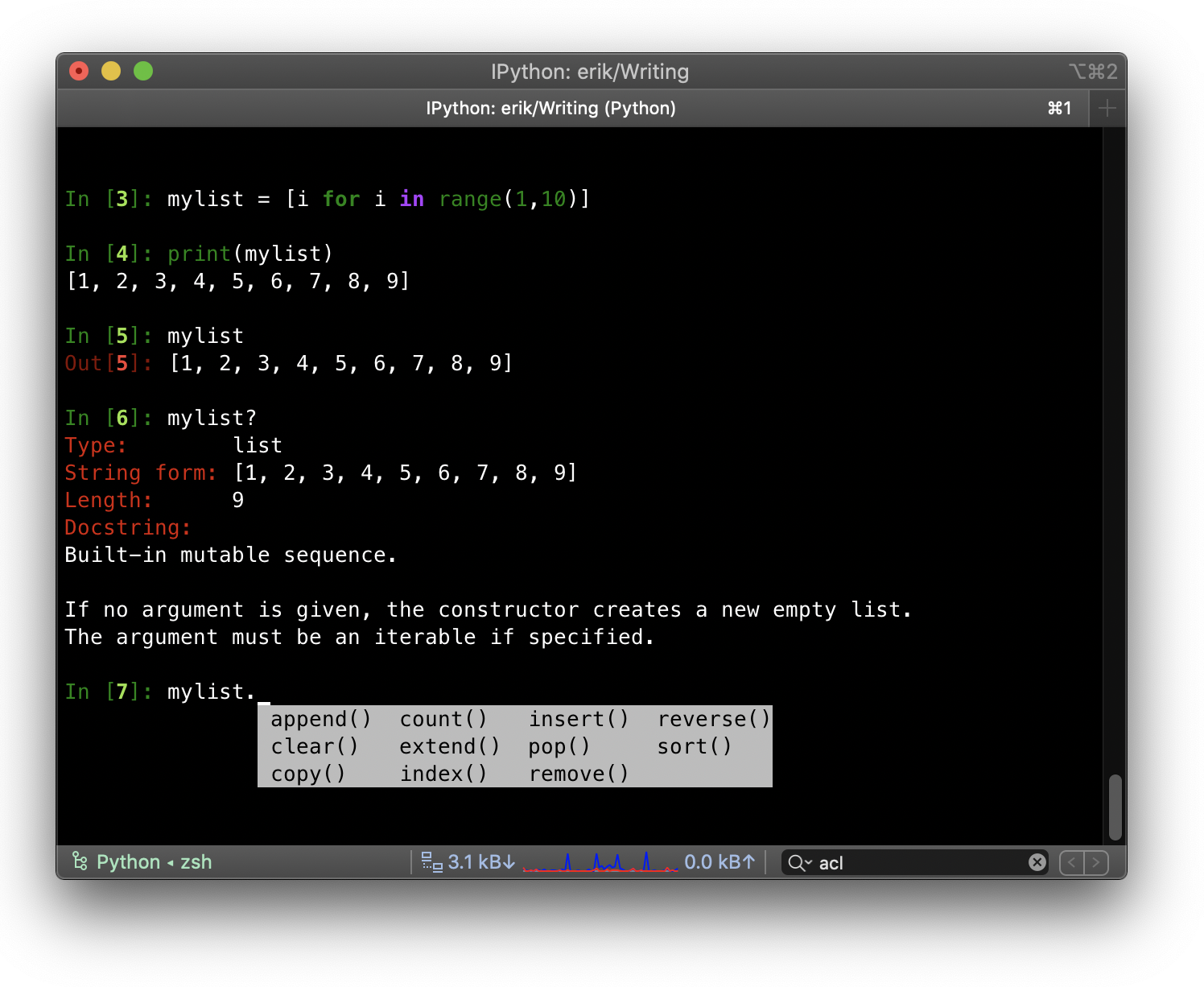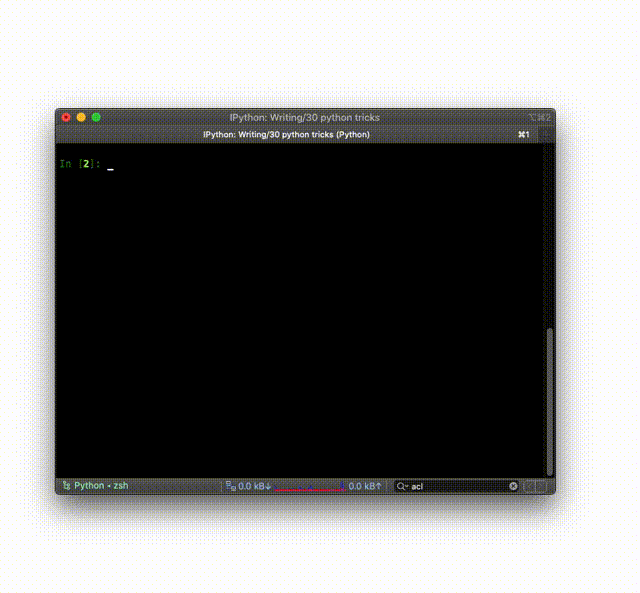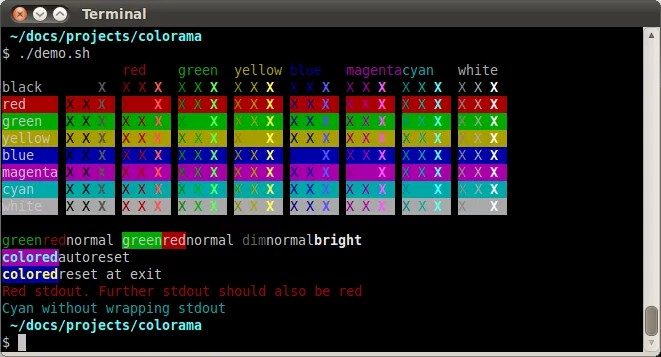# 30个 Python3 的最佳实践，技巧和窍门

huatechinfo

0收藏

1、使用 Python3

2、检查所需的最低 Python 版本

``````if not sys.version_info > (2, 7):
# berate your user for running a 10 year
# python version
elif not sys.version_info >= (3, 5):
# because you're using 3.5 features``````

3. Use IPythonIPython 其实就是升级版的 shell，单单是自带的自动补全功能就值得你使用它了。不过它的优势不止这些，它那些如魔法般的内置命令行也让是我爱使用它的原因。这些命令有：

• %cd—修改当前工作路径
• %edit—打开编辑器，并在关闭时执行你刚刚输入的代码
• %env—显示当前环境变量
• %pip install [pkgs]—在不离开 Shell 的情况下安装包
• %time and %timeit—为 Python 代码计时
如果你想了解更多的命令，可以参考这个网站：

``pip3 install ipython``

4、列表表达式

``[ expression for item in list if conditional ]``

``````mylist = [i for i in range(10)]
print(mylist)
# 0 1 2 3 4 5 6 7 8 9``````

在这条命令里还可以使用表达式（expression），所以也可以做一些数学运算：

``````squares = [x**2 for x in range(10)]
print(squares)
# [0, 1, 4, 9, 16, 25, 36, 49, 64, 81]``````

``````def some_function(a):
return (a + 5) / 2

my_formula = [some_function(i) for i in range(10)]
print(my_formula)
# [2.5, 3.0, 3.5, 4.0, 4.5, 5.0, 5.5, 6.0, 6.5, 7.0]``````

``````filtered = [i for i in range(20) if i%2==0]
print(filtered)
# [0, 2, 4, 6, 8, 10, 12, 14, 16, 18]``````

5、检查你的对象占用了多少内存

``````import sys

mylist = range(0, 10000)
print(sys.getsizeof(mylist))
# 48``````

``````import sys

myreallist = [x for x in range(0, 10000)]
print(sys.getsizeof(myreallist))
# 87632``````

6、返回多个值

Python 的函数可以同时返回多个值，也并不需要使用 dictionary，list 或是类这样的数据结构。它的工作机制是这样的

``````def get_uset(id):
# 从数据库中获取用户
# ...
return name,birthdate

name,birthdate = get_user(4)``````

7、使用 数据类
Python 从 3.7 开始提供数据类功能。这种功能与常规的类以及其他类似的功能（返回多个值的函数以及 dictionary）相比较，有以下优势：

• 因为数据类已经自带了__eq__功能，故而你可以直接进行数据类的对比
• 数据类自带__repr__，你也可以直接在 debug 时打印出一个数据类
• 数据类型需要你输入提示，这样 bug 量会大大减少

``````from dataclasses import dataclass

@dataclass
class Card:
rank: str
suit: str

card = Card("Q", "hearts")

print(card == card)
# True

print(card.rank)
# 'Q'

print(card)
Card(rank='Q', suit='hearts')``````

8、在位置不变的情况下交换变量

``````a = 1
b = 2
a, b = b, a
print(a)
# 2
print(b)
# 1``````

9、合并 dictionary（Python 3.5+）

``````dict1 = { 'a': 1, 'b': 2 }
dict2 = { 'b': 3, 'c': 4 }
merged = { **dict1, **dict2 }
print (merged)
# {'a': 1, 'b': 3, 'c': 4}``````

10、把字符串变成标题形式

``````mystring = "10 awesome python tricks"
print(mystring.title())
'10 Awesome Python Tricks'``````

11、把字符串分割成 list

``````mystring = "The quick brown fox"
mylist = mystring.split(' ')
print(mylist)
# ['The', 'quick', 'brown', 'fox']``````

12、将一个字符串列表变成字符串

``````mylist = ['The', 'quick', 'brown', 'fox']
mystring = " ".join(mylist)
print(mystring)
# 'The quick brown fox'``````

``mylist.join(" ")``

13、Emoji

``pip install emoji``

``````import emoji
result = emoji.emojize('Python is :thumbs_up:')
print(result)
# 'Python is 👍'

# You can also reverse this:
result = emoji.demojize('Python is 👍')
print(result)
# 'Python is :thumbs_up:'``````

14、list 切片 (重要）
list 切片的基本使用形式如下：

``a[start:stop:step]``

Start, Stop 和 Step 都是可选参数。如果你没有定义，它们就会按照如下规则分配默认值：

• start = 0
• end = 方括号里面字符串的最后一个字符
• step = 1
``````# 我们可以轻松地从创建新列表
# 列表的前两个元素：
first_two = [1, 2, 3, 4, 5][0:2]
print(first_two)
# [1, 2]

# 如果我们使用 step 值为2，
# 我们可以跳过第二个数字
# like this:
steps = [1, 2, 3, 4, 5][0:5:2]
print(steps)
# [1, 3, 5]

# 这也适用于字符串。 在Python中，
# 您可以将字符串视为
# letters:
mystring = "abcdefdn nimt"[::2]
print(mystring)
# 'aced it'``````

15、翻转字符串和 list

``````revstring = "abcdefg"[::-1]
print(revstring)
# 'gfedcba'

revarray = [1, 2, 3, 4, 5][::-1]
print(revarray)
# [5, 4, 3, 2, 1]``````

16、展示小猫的图片

``pip3 install Pillow````````from PIL import Image

im = Image.open("kittens.jpg")
im.show()
print(im.format, im.size, im.mode)
# JPEG (1920, 1357) RGB``````Pillow 能做的可不止这些。它可以对图片进行分析、裁剪、过滤、增强、变形等等。如果想要更深入的了解，可以去看一下它的文档：

17、使用  map()
Python 的内置函数之一就是 map()。map() 的语法如下：

``````def upper(s):
return s.upper()

mylist = list(map(upper, ['sentence', 'fragment']))
print(mylist)
# ['SENTENCE', 'FRAGMENT']

# 转换的字符串表示形式
# 整数列表中的数字。
list_of_ints = list(map(int, "1234567")))
print(list_of_ints)
# [1, 2, 3, 4, 5, 6, 7]``````

18、从 list 或是 string 中获取 unique 元素

``````mylist = [1, 1, 2, 3, 4, 5, 5, 5, 6, 6]
print (set(mylist))
# {1, 2, 3, 4, 5, 6}

# 由于字符串可以被视为
# 字母列表，您还可以通过以下方式从字符串中获取唯一字母：
print (set("aaabbbcccdddeeefff"))
# {'a', 'b', 'c', 'd', 'e', 'f'}``````

19、找到高频值

``````test = [1, 2, 3, 4, 2, 2, 3, 1, 4, 4, 4]
print(max(set(test), key = test.count))
# 4``````

• max() 会返回 list 中的最高值。而 key 可以利用一个输入（如本例中的 test.count）来确定你要排序的方式。这个函数会应用于前面可迭代对象的每一项。
• test.count 是 list 的内置函数。我们给它一个输入，它会统计那个输入的出现次数。test.count(1) 就会返回 2，test.count(4) 就会返回 4。
• set(test) 会返回 unique 值，也就是 {1, 2, 3, 4}

20、创建进度条

``pip3 install progress``

``````from progress.bar import Bar

bar = Bar('Processing', max=20)
for i in range(20):
# Do some work
bar.next()
bar.finish()``````21、在交互 shell 中使用「_」

``````In : 3 * 3
Out: 9
In : _ + 3
Out: 12``````

Python shell 中这条命令也可以用。IPython shell 中你也可以用 Out[n] 来获得 In[n] 的输出。比如说，在上面的例子中，Out 就会返回 9。

22、快速创建一个网页服务器

``python3 -m http.server``

23、多行字符串

``````s1 = """Multi line strings can be put
between triple quotes. It's not ideal

print (s1)
# Multi line strings can be put
#         between triple quotes. It's not ideal
#         when formatting your code though

s2 = ("You can also concatenate multiple\n" +
"strings this way, but you'll have to\n"
"explicitly put in the newlines")

print(s2)
# You can also concatenate multiple
# strings this way, but you'll have to
# explicitly put in the newlines``````

24、条件赋值的三元运算符

``[on_true] if [expression] else [on_false]``

``x = "Success!" if (y == 2) else "Failed!"``

25、统计出现数

``````from collections import Counter

mylist = [1, 1, 2, 3, 4, 5, 5, 5, 6, 6]
c = Counter(mylist)
print(c)
# Counter({1: 2, 2: 1, 3: 1, 4: 1, 5: 3, 6: 2})

# 它也适用于字符串：
print(Counter("aaaaabbbbbccccc"))
# Counter({'a': 5, 'b': 5, 'c': 5})``````

26、比较符链（chaining of comparison operators）
Python 里，你可以把比较符连接成一条链，这样代码会更有可读性，而且更简洁。

``````x = 10

# 代替：
if x > 5 and x < 15:
print("Yes")
# yes

# You can also write:
if 5 < x < 15:
print("Yes")
# Yes``````

27、加一些色彩Colorama 中 Jonathan Hartley 的截屏

``````from colorama import Fore, Back, Style

print(Fore.RED + 'some red text')
print(Back.GREEN + 'and with a green background')
print(Style.DIM + 'and in dim text')
print(Style.RESET_ALL)
print('back to normal now')``````

28、日期计算
python-dateutil 模块对标准的 datatime 模块做了很大的扩充。先下载一下这个模块：

``pip3 install python-dateutil``

``````from dateutil.parser import parse

logline = 'INFO 2020-01-01T00:00:01 Happy new year, human.'
timestamp = parse(logline, fuzzy=True)
print(timestamp)
# 2020-01-01 00:00:01``````

29、整除
Python 2 中，除号（/）默认为整除，除非其中一个是浮点数。所以你会得到这样的结果：

``````# Python 2
5 / 2 = 2
5 / 2.0 = 2.5``````

``````Python 3
5 / 2 = 2.5
5 // 2 = 2``````

30、使用 chardet 检测 Charset

``pip install chardet``

``````chardetect somefile.txt
somefile.txt: ascii with confidence 1.0``````帖子
视频
声望
粉丝
相关问题
社区精华内容